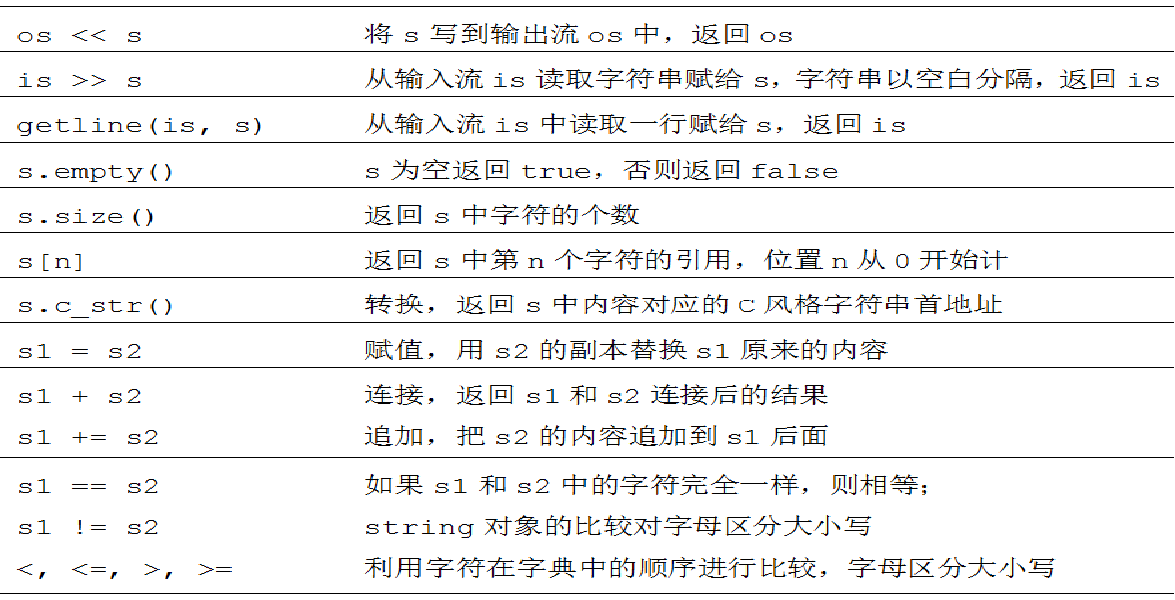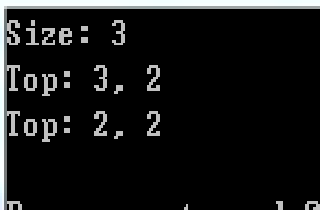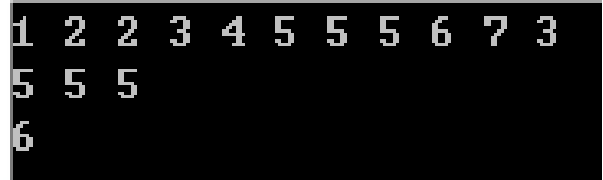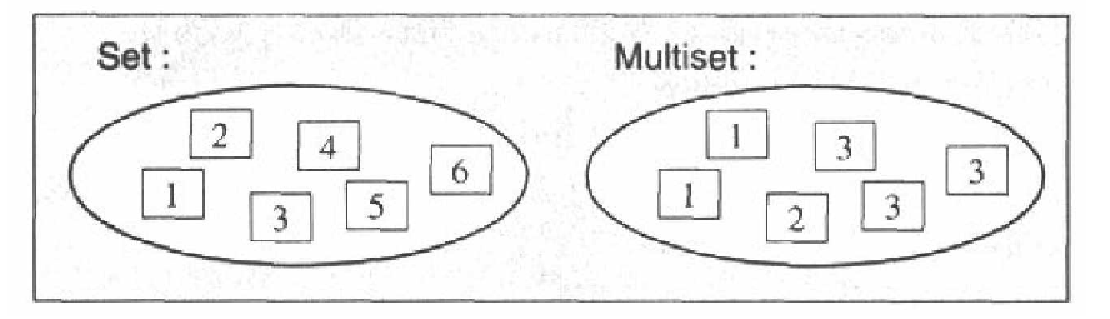## 山东农业大学ACM学习，第二周学习心得_大大大太阳chen的博客-程序员宅基地

STL的简单应用：string stack queue vector sort priority_queue unique upper_bound lower_bound set和multiset map和multimap简单应用

1.string (C++的字符串类型)
.string 表示可变长度的字符序列

…string 类支持字符串对象的各种操作

…使用string 类要包含头文件empty()函数判断string对象是否为空
size()函数返回string对象的长度，即对象中字符的个数

#include
using namespace std;
int main()
{
string s1, s2;
string s3 = “Hello, World!”;
string s4("I am ");
s2 = “Today”;
s1 = s3 + " " + s4;
s1 += " 5 “;
cout << s1 + s2 + “!” <<endl;
cout <<“Length of s1 is :” << s1.size() << endl;
for (int i = 0; i < s1.size(); ++i)
cout << s1[i] <<” ";
}
/*
Hello, World! I am 5 Today!
Length of s1 is :22
H e l l o , W o r l d ! I a m 5
Process returned 0 (0x0) execution time : 0.167 s
Press any key to continue.
*/

1.栈（stack）
stack是一种先进后出(First In Last Out, FILO)的数据结构，它只有一个出口，只能操作最顶端元素。

stack<data_type> stack_name;

empty() – 返回bool型，表示栈内是否为空 (s.empty() )
size() – 返回栈内元素个数 (s.size() )
top() – 返回栈顶元素值 (s.top() )
pop() – 移除栈顶元素(s.pop(); )
push(data_type a) – 向栈压入一个元素 a(s.push(a); )
**2.**队列（queue）
queue是一种先进先出(First In First Out, FIFO)的数据结构，从底端加入元素，从顶端取出元素

empty() – 返回bool型，表示queue是否为空 (q.empty() )
size() – 返回queue内元素个数 (q.size() )
front() – 返回queue内的下一个元素 (q.front() )
back() – 返回queue内的最后一个元素(q.back() )
pop() – 移除queue中的一个元素(q.pop(); )
push(data_type a) – 将一个元素a置入queue中(q.push(a); )
3.vector动态数组

empty() – 返回bool型，表示vector是否为空 (v.empty() )
size() – 返回vector内元素个数 (v.size() )
push_back(data_type a) 将元素a插入最尾端
pop_back() 将最尾端元素删除
v[i] 类似数组取第i个位置的元素(v )
4.sort排序（超级经典，个人很喜欢用）

sort(begin, end);
sort(begin, end, cmp);

int num[] = {1,5,6,2,9};
1) sort(num, num + 5);//默认从小到大排序num[] = {1,2,5,6,9};
2) bool cmp(int a, int b){
return a > b;
}
sort(num, num + 5, cmp); //num[] = {9,6,5,2,1};

#include<bits/stdc++.h>
using namespace std;

int main(){
sync_with_stdio(false);
int a;
vector b;
for(int i=0;i<5;i++)
{
cin>>a[i];
b.push_back(a[i]);
}
sort(a,a+5);
sort(b.begin(),b.end());
for(int i=0;i<5;i++)
cout<<a[i]<<" “;
cout<<endl;
for(int i=0;i<5;i++)
cout<<b[i]<<” ";
return 0;
}

5.优先序列（priority_queue）

q.push(elem) 将元素elem置入优先队列
q.top() 返回优先队列的下一个元素
q.pop() 移除一个元素
q.size() 返回队列中元素的个数
q.empty() 返回优先队列是否为空

int main(){
priority_queue s;
coord a;
a.x = 3, a.y = 2;
s.push(a);
a.x = 1, a.y = 2;
s.push(a);
a.x = 2, a.y = 2;
s.push(a);
cout << "Size: " << s.size() << endl;
cout << "Top: " << s.top().x << ", " << s.top().y << endl;
s.pop();
cout << "Top: " << s.top().x << ", " << s.top().y << endl;
return 0;
}1.去重unique
iterator unique(iterator begin,iterator);
iterator unique(iterator begin,iterator end,bool MyFunc);

bool next_permutation(begin, end);

bool prev_permutation(begin, end);

end为最后一个元素的下一个位置。

2.upper_bound 和 lower_bound的使用方法
upper_bound(begin, end, value);

lower_bound(begin, end, value);

num[] = {1,2,2,3,4,5};
lower_bound(num, num + 6, 2)为num + 1
upper_bound(num, num + 6, 2)为num + 3 //注意理解这种说法

#include<bits/stdc++.h>
using namespace std;

int main(){
sync_with_stdio(false);
int a = {1,3,5,2,4,6,7,5,2,5};
sort(a, a + 10);
for (int i = 0; i < 10; ++i) cout<<a[i]<<" “; //排序从小到大
int *s, *e;
s = lower_bound(a, a + 10, 5); // *此时这个s所指地址为排序后元素的地址 此时的s为第六个元素的地址
e = upper_bound(a, a + 10, 5); //此时e的地址为第九个元素的地址
cout<<e-s<<endl; //地址-地址得数字3（中间隔了三个元素所以是3）
while(s!= e){
cout<<*s++<<” "; //重新排序后三个5连着排在一起所以输出三个5
}
cout<<endl;
cout<<*e<<endl;
return 0; //e为第九个元素的地址，即为数6的地址，所以输出6
}1.set 和 multiset
set 和 multiset会根据特定的排序准则，自动将元素排序，两者的不同之处在于multiset可以允许元素重复而set不允许元素重复。‘set和multiset 简单应用

s.insert(elem) – 安插一个elem副本，返回新元素位置。
s.erase(elem) – 移除与elem元素相等的所有元素，返回被移除 的元素个数。
s.erase(pos) – 移除迭代器pos所指位置上的元素，无返回值。
s.clear() – 移除全部元素，将整个容器清空。

multiset :: iterator pos;
for(pos = s.begin(); pos != s.end(); pos++)
… …
set和multiset 简单应用

s.size() – 返回容器大小。
s.empty() – 返回容器是否为空。
s.count(elem) – 返回元素值为elem的元素的个数。
s.lower_bound(elem) – 返回 元素值>= elem的第一个元素位置。
s.upper_bound(elem) – 返回元素值 > elem的第一个元素的位置。

s.begin() – 返回一个双向迭代器，指向第一个元素。
s.end() – 返回一个双向迭代器，指向最后一个元素的下一 个位置

2.map和multimap

m.size() 返回容器大小
m.empty() 返回容器是否为空
m.count(key) 返回键值等于key的元素的个数
m.lower_bound(key) 返回键值等于key的元素的第一个可安插 的位置
m.upper_bound(key) 返回键值等于key的元素的最后一个可安 插的位置

map和multimap简单应用

m.begin() 返回一个双向迭代器，指向第一个元素。
m.end() 返回一个双向迭代器，指向最后一个元素的下一个 位置。
m.clear() 讲整个容器清空。
m.erase(elem) 移除键值为elem的所有元素，返回个数，对 于map来说非0即1。
m.erase(pos) 移除迭代器pos所指位置上的元素。

m[key] = value；

STL简单运用，现在只学了一小部分，从string字符串开始引出对于字符串的相关操作，栈，队列，排序，优先队列都是用来排序。而去重和位置寻找问题的解决思路也相继出现。简单的排列后又学了自定义排列顺序的排列方法。

### CTF中的md5弱类型（ALL_IN_ONE）_ctf all in one-程序员宅基地

CTF中的md5文章目录第一种情况，md5弱类型比较第二种情况，md5强类型比较第三种情况，md5碰撞第四种情况，sql语句md5值暴破其他特殊的hash值(md4、crc32......)第一种情况，md5弱类型比较if(md5($_GET['a'])==md5($_GET['b'])){var_dump(\$flag);}因为是if的判断条件是两个数弱类型相等，就可以利用hash比较缺陷去绕过比如var_dump("0e12345"=="0e66666");//truevar_dum_ctf all in one

### linux rz scp,Linux命令学习(5)-sftp,scp,rz,sz-程序员宅基地

sftp###命令行模式的安全文件传输协议。通过ssh的通道进行传输的，默认端口为22常用选项：-o : 回话配置(session-config), 可以用man查看详细介绍。我主要是用来配置远程连接的端口号，因为我的主机的远程端口号不是默认的22.sftp -oPort=333 [email protected]模式下常用的命令：sftp_cmd.pngscp###知道远程目录文件的情况下可使..._linux rz是用什么端口传的

### TensorRT用自带trtexec实现onnx转engine的用法说明_trtexec在哪-程序员宅基地

TensorRT自带的trtexec在bin目录下，是一个可执行文件。运行./trtexec -h其中给出了 model options、build options、 inference options和system options等。上次我们使用TensorRT的pyhton API进行序列化模型和前向推理，这次介绍使用trtexec转模型。1.从固定尺寸的onnx转cudaEngine./trtexec --explicitBatch --onnx=./resnet50.onnx --sa_trtexec在哪

### VUE：如何实现组件异步加载？_vue如何异步加载组件-程序员宅基地

1. 什么是异步组件？即只在组件需要渲染的时候进行加载渲染并缓存。2. 为什么需要异步加载组件？当项目功能越来越多，所包含的子组件也越来越多，导致页面加载，访问速度过慢，所以需要优化页面加载的性能。3. 异步加载组件方法可以使用懒加载，即 () => import ( 地址)关于路由懒加载：VUE：实现路由懒加载使用require// 全局组件注册Vue.component('Home, function (resolve) { // 这个特殊的 require_vue如何异步加载组件

### java实现轮询和加权轮询-程序员宅基地

1. 一般轮询算法服务器类package com.sosop.roundRobin;public class Server { private String ip; private int weight; public Server(String ip) { super(); this.ip = ip; } public Server(..._java中两个数字轮询

### JEECG Online Coding 开发操作图解_jeecg官网online coding-程序员宅基地

JEECG Online Coding 开发操作图解一、 单表智能表单1. 创建数据表单（界面如下图）创建单表时要有id主键 设置不允许空值、不显示、不查询并且主键只有id数据表单类型为单表2. 录入表单信息后，保存表单，同步到数据库同步数据库3. 进入数据列表进行表单维_jeecg官网online coding

### ognl-程序员宅基地

ognl：对象图导航语言,表达式；el表达式：获取域对象中的值。在Struts2中主要用来获取值栈中的内容。ognl不是Struts2中的一部分，它是完全独立存在的，如果想使用ognl需要导入ognl的jar包。实现功能： 1、支持对象方法调用，如xxx.doSomeSpecial()； 2、支持类静态的方法调用和值访问，表达式的格式: @[类全名（包括包路径）]@..._ognl:lisenteres